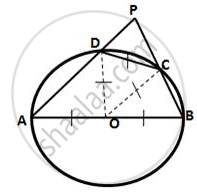Share

# In a Circle, with Centre O, a Cyclic Quadrilateral Abcd is Drawn with Ab as a Diameter of the Circle and Cd Equal to Radius of the Circle. If Ad and Bc Produced Meet at Point P; Show that ∠Apb = 60°. - Mathematics

Course

#### Question

In a circle, with centre O, a cyclic quadrilateral ABCD is drawn with AB as a diameter of the circle and CD equal to radius of the circle. If AD and BC produced meet at point P; show that ∠APB = 60°.

#### SolutionJoin OD and OC.
ΔOCD, OD = OD= CD
∴ ΔOCD is an equilateral triangle
∴ ∠ODC =60°
(pair of opposite angles in cyclic quadrilateral are supplementary)
⇒ ∠ODA + 60° + ∠ABP =180°
⇒ ∠OAD + ∠ABP = 90° ( ∵  OA = OD)
⇒ ∠PAB = ∠ABP =120°
By angle sum property of ΔPAB,
∴ ∠APB = 180° - ∠PAB -∠ABP =180° -120°  =60°

Is there an error in this question or solution?